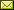## Generating Randomness from a Computable, Non-random Sequence of Qubits

 Tejas Bhojraj (Department of Mathematics, University of Wisconsin-Madison)

 Nies and Scholz introduced the notion of a state to describe an infinite sequence of qubits and defined quantum-Martin-Lof randomness for states, analogously to the well known concept of Martin-Löf randomness for elements of Cantor space (the space of infinite sequences of bits). We formalize how 'measurement' of a state in a basis induces a probability measure on Cantor space. A state is 'measurement random' (mR) if the measure induced by it, under any computable basis, assigns probability one to the set of Martin-Löf randoms. Equivalently, a state is mR if and only if measuring it in any computable basis yields a Martin-Löf random with probability one. While quantum-Martin-Löf random states are mR, the converse fails: there is a mR state, x which is not quantum-Martin-Löf random. In fact, something stronger is true. While x is computable and can be easily constructed, measuring it in any computable basis yields an arithmetically random sequence with probability one. I.e., classical arithmetic randomness can be generated from a computable, non-quantum random sequence of qubits.

In Bob Coecke and Matthew Leifer: Proceedings 16th International Conference on Quantum Physics and Logic (QPL 2019), Chapman University, Orange, CA, USA., 10-14 June 2019, Electronic Proceedings in Theoretical Computer Science 318, pp. 1–12.
Published: 1st May 2020.

 ArXived at: https://dx.doi.org/10.4204/EPTCS.318.1 bibtex PDF
References in reconstructed bibtex, XML and HTML format (approximated).
 Comments and questions to:eptcs@eptcs.org For website issues:webmaster@eptcs.org